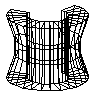library: libGraf3d#include "TPCON.h"

# TPCON

class description - source file - inheritance tree (.pdf)

## class TPCON : public TShape

Inheritance Chart:
 TObject
<-
TNamed
 TAttLine
 TAttFill
 TAtt3D
<-
TShape
<-
TPCON
<-
 TPGON
```
protected:
virtual void FillTableOfCoSin(Double_t phi, Double_t angstep, Int_t n) const
virtual void MakeTableOfCoSin() const
virtual void SetPoints(Double_t* points) const
virtual Bool_t SetSegsAndPols(TBuffer3D& buffer) const

public:
TPCON()
TPCON(const char* name, const char* title, const char* material, Float_t phi1, Float_t dphi1, Int_t nz)
TPCON(const TPCON&)
virtual ~TPCON()
static TClass* Class()
virtual void DefineSection(Int_t secNum, Float_t z, Float_t rmin, Float_t rmax)
virtual Int_t DistancetoPrimitive(Int_t px, Int_t py)
virtual const TBuffer3D& GetBuffer3D(Int_t reqSections) const
virtual Float_t GetDhi1() const
virtual Float_t* GetDz() const
virtual Int_t GetNdiv() const
virtual Int_t GetNumberOfDivisions() const
virtual Int_t GetNz() const
virtual Float_t GetPhi1() const
virtual Float_t* GetRmax() const
virtual Float_t* GetRmin() const
virtual TClass* IsA() const
TPCON& operator=(const TPCON&)
virtual void SetNumberOfDivisions(Int_t p)
virtual void ShowMembers(TMemberInspector& insp, char* parent)
virtual void Sizeof3D() const
virtual void Streamer(TBuffer& b)
void StreamerNVirtual(TBuffer& b)
```

### Data Members

```
protected:
Double_t* fSiTab  ! Table of sin(fPhi1) .... sin(fPhil+fDphi1)
Double_t* fCoTab  ! Table of cos(fPhi1) .... cos(fPhil+fDphi1)
Float_t fPhi1   lower phi limit
Float_t fDphi1  range in phi
Int_t fNdiv   number of divisions
Int_t fNz     number of z segments
Float_t* fRmin   [fNz] pointer to array of inside radiuses
Float_t* fRmax   [fNz] pointer to array of outside radiuses
Float_t* fDz     [fNz] pointer to array of half lengths in z
```

## Class Description

` ```` PCON is a polycone. It has the following parameters:

- name       name of the shape
- title      shape's title
- material  (see TMaterial)
- phi1       the azimuthal angle phi at which the volume begins (angles
are counted counterclockwise)
- dphi       opening angle of the volume, which extends from
phi1 to phi1+dphi
- nz         number of planes perpendicular to the z axis where
the dimension of the section is given -- this number
should be at least 2
- rmin       array of dimension nz with minimum radius at a given plane
- rmax       array of dimension nz with maximum radius at a given plane
- z          array of dimension nz with z position of given plane
```

TPCON()
``` PCON shape default constructor
```

TPCON(const char *name, const char *title, const char *material, Float_t phi1, Float_t dphi1, Int_t nz) : TShape(name, title,material)
``` PCON shape normal constructor

Parameters of the nz positions must be entered via TPCON::DefineSection.
```

void MakeTableOfCoSin() const

~TPCON()
``` PCON shape default destructor
```

void DefineSection(Int_t secNum, Float_t z, Float_t rmin, Float_t rmax)
``` Defines section secNum of the polycone

- rmin  radius of the inner circle in the cross-section

- rmax  radius of the outer circle in the cross-section

- z     z coordinate of the section
```

Int_t DistancetoPrimitive(Int_t px, Int_t py)
``` Compute distance from point px,py to a PCON

Compute the closest distance of approach from point px,py to each
computed outline point of the PCON.
```

void FillTableOfCoSin(Double_t phi, Double_t angstep,Int_t n) const
``` Fill the table of cos and sin to prepare drawing
```

void SetNumberOfDivisions (Int_t p)

void SetPoints(Double_t *points) const
``` Create PCON points
```

void Sizeof3D() const
``` Return total X3D needed by TNode::ls (when called with option "x")
```

void Streamer(TBuffer &b)
``` Stream a class object
```

const TBuffer3D& GetBuffer3D(Int_t reqSections) const

Bool_t SetSegsAndPols(TBuffer3D & buffer) const

### Inline Functions

```              Int_t GetNumberOfDivisions() const
Float_t GetPhi1() const
Float_t GetDhi1() const
Int_t GetNz() const
Float_t* GetRmin() const
Float_t* GetRmax() const
Float_t* GetDz() const
Int_t GetNdiv() const
TClass* Class()
TClass* IsA() const
void ShowMembers(TMemberInspector& insp, char* parent)
void StreamerNVirtual(TBuffer& b)
TPCON TPCON(const TPCON&)
TPCON& operator=(const TPCON&)
```